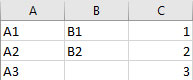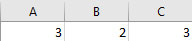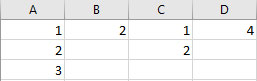# ANOVA HelpANOVA (Analysis of Variances) is used to determine which factors have a significant effect on a variable.   The program has the following options:

• 1 to 5 factors
• Random and/or fixed factors
• Crossed, nested or mixed designs

To access this feature, select “ANOVA” in the “Analysis” panel in the SPC for Excel ribbon.

The full model is used (e.g, all factors and interactions).  The ANOVA must be set up before the analysis can be done.  The data entry requirements depend on the type of design you have.

The links below provide more help on ANOVA.

The data entry for the three types (crossed, nested and mixed) are shown below.

### Data Entry for ANOVA

The design must be setup to start.   The program requires this to occur for the analysis portion to be run.

Crossed Design Setup

Factor A is crossed with factor B if each level of Factor A appears with each level of Factor B and vice-versa.  The software denotes crossed factors by A*B.  To setup a crossed design, enter the factor names with the levels under each factor into a spreadsheet.  An example for three factors is shown below.  The factors are A, B and C.  The levels are listed under each factor and can be numbers or strings.Nested Design Setup

Factor B is nested with factor A if each level of B occurs in conjunction with only one level of A.  The software denotes a nested factor as B(A).  To setup a nested design, enter the factor names with the number of levels under each name.  The numbers must be integers.  An example for three factors is shown below.  You will have the option to add names to the levels under each factor.  These must be entered into the spreadsheet.Mixed Design Setup

A mixed design contains both crossed and nested factors.  To setup a mixed design, enter the factors names into a spreadsheet.  Under the first factor, list the actual levels (like in the crossed design setup above).  Under the remaining factors, list the levels if the factor is crossed; list the number of levels if the factor is nested.  A four factor example is shown below where factors B and D are nested.  You will have the option to add names to the levels for the nested factors.  These must be entered into the spreadsheet.